出租广告位,需要合作请联系站长

+关注

2020-06(12)

2020-07(30)

2020-08(30)

2020-09(66)

2020-10(79)

## Python-基于卷积神经网络斑马线识别分类，准确率高达99%## 神经网络的结构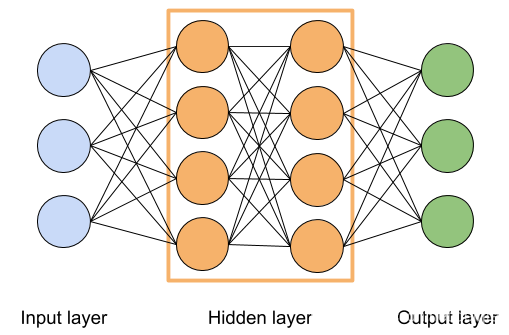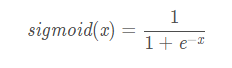sigmoid函数图像如下图所示。当 x > > 0 ​ x>>0​ x>>0​时， f ( x ) ​ f(x)​ f(x)​无限逼近于1；当 x < < 0 ​ x<<0​ x<<0​时， f ( x ) ​ f(x)​ f(x)​无限逼近于0；当 x = 0 ​ x=0​ x=0​时， f ( x ) = 0.5 ​ f(x)=0.5​ f(x)=0.5​。

## 搭建模型

##### 一、引入基本库

• Windows10系统
• Python–3.7.3
• tensorflow–2.3
• OpenCV–4.5.1
``````import tensorflow as tf
import matplotlib.pyplot as plt
import cv2 as cv
import pandas as pd
import numpy as np
import os
import shutil
from keras.models import Sequential, load_model
from keras import optimizers
from keras.preprocessing.image import ImageDataGenerator
from keras.layers import Conv2D, MaxPooling2D, Flatten, Dense
``````

`Conv2D :卷积`
`MaxPooling2D: 最大池化`
`Flatten: 从卷积层到全连接层的过渡（卷积），扁平数据`
`Dense: 全连接`

##### 二、数据集准备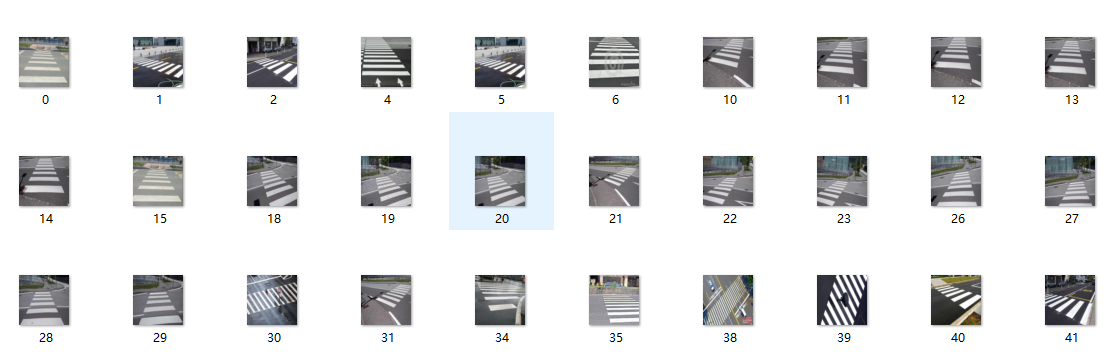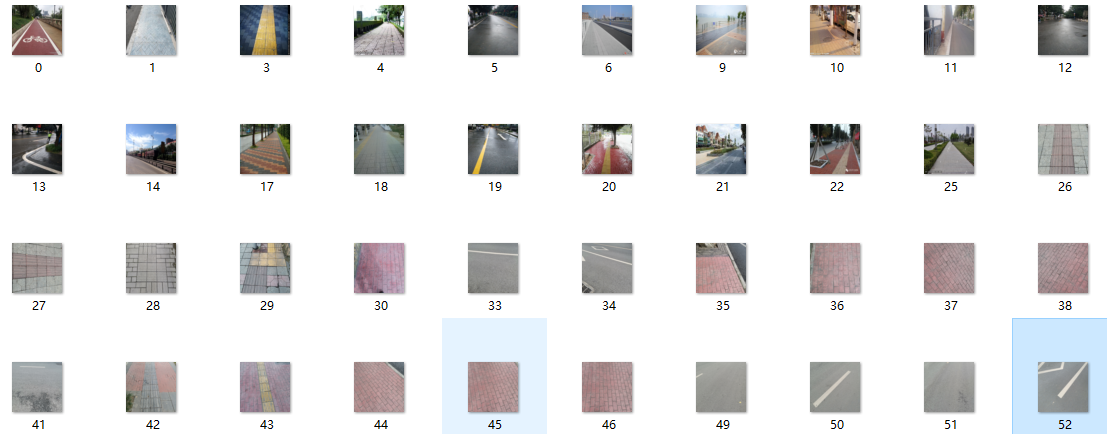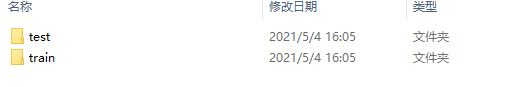ImageDataGenerator图像生成器他会根据train和test的子文件信息来给予标签；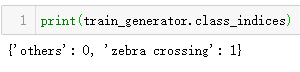``````#简单的二分类
train_dir = 'G:\\深度学习\\Zebra\\Zebra\\train'#训练集文件
val_dir = 'G:\\深度学习\\Zebra\\Zebra\\test'  #验证集文件
train_datagen = ImageDataGenerator(rescale=1/255)
train_generator = train_datagen.flow_from_directory(train_dir, (50, 50), batch_size=20, class_mode='binary', shuffle=True)
validation_datagen = ImageDataGenerator(rescale=1/255)
validation_generator = validation_datagen.flow_from_directory(val_dir, (50, 50), batch_size=20, class_mode='binary')
``````
##### 三、模型搭建

``````model = Sequential()
model.add(Conv2D(32, (3, 3),activation='relu', input_shape=(50, 50, 3)))
model.add(MaxPooling2D((2, 2)))
model.add(Conv2D(64, (3, 3), activation='relu'))
model.add(MaxPooling2D((2, 2)))
model.add(Conv2D(128, (3, 3), activation='relu'))
model.add(MaxPooling2D((2, 2)))
model.add(Conv2D(128, (3, 3), activation='relu'))
model.add(MaxPooling2D((2, 2)))
model.add(Flatten())
model.add(Dense(512, activation='relu'))
model.add(Dense(1, activation='sigmoid'))
model.summary()
``````
##### 四、模型训练
``````model.compile(loss='binary_crossentropy',#二分类，使用binary_crossentropy损失函数
optimizer=optimizers.Adam(), #Adam优化器
metrics=['acc']
)
history = model.fit_generator(train_generator,
steps_per_epoch=17,
epochs=25,
validation_data=validation_generator,
validation_steps=6)

``````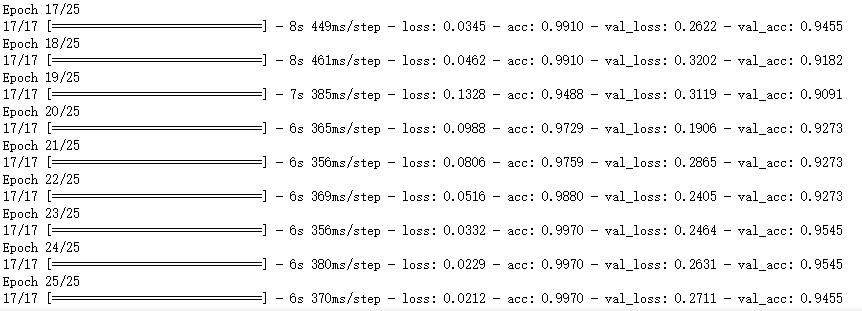##### 五、总结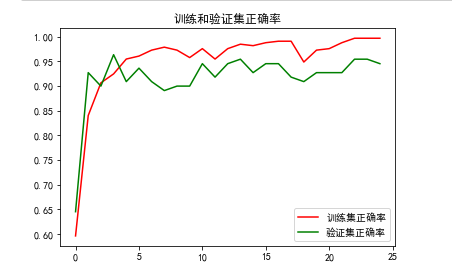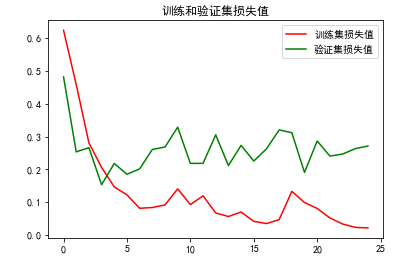train loss 不断下降，test loss趋于不变，说明网络过拟合;

• 改变模型，模型太大，太深，参数太多难以收敛，建议使用简单的模型
• 增加数据集
• 增大batch_size
• 添加正则
• dropsort 减少参数
这些方法都可以在抑制模型过拟合，但是最好的方法是改变模型增加数据集

15 0

pdf(new) 更多>